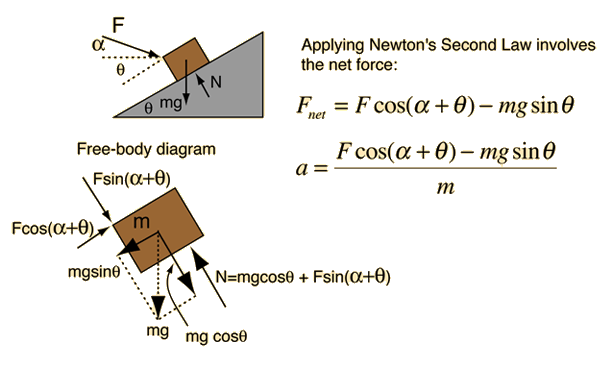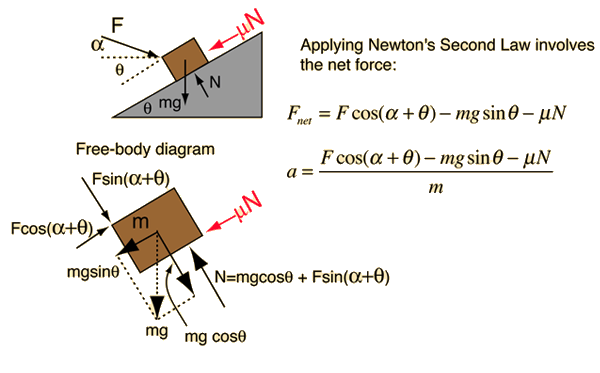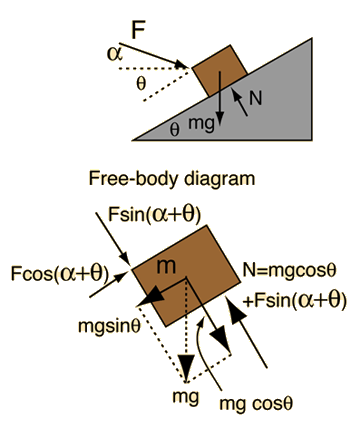# Force on Inclined Mass### Calculation

Index

Newton's laws

Standard mechanics problems

 HyperPhysics***** Mechanics R Nave
Go Back

# Force on Inclined Mass### Remove friction

Index

Newton's laws

Standard mechanics problems

 HyperPhysics***** Mechanics R Nave
Go Back

# Force on Inclined MassApplication of Newton's second law to mass on incline.
Given a mass of kg on an incline of angle degrees, if an external force of Newtons is applied at an angle degrees above the horizontal, then the acceleration up the incline will be

### Acceleration =m/s2

In this case, a negative acceleration implies that the force is insufficient to push the mass up the incline, and it is sliding downward.